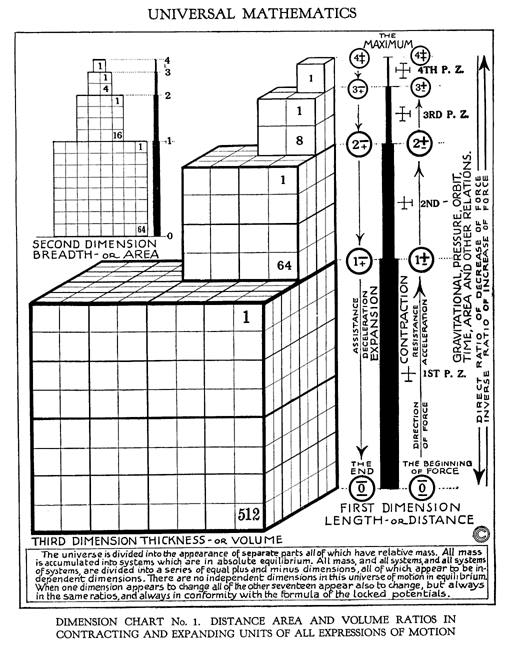# Table 12.02 - Length Area and Volume MathFigure 12.09 - Dimensions and Their Relationships

In graphic Figure 12.09 - Dimensions and Relationships it is clear:

Relative Volume

Accumulating Dispersing

4+ = 1/8 of 3+ or 3+ = 8 X 4+ or 81

3+ = 1/8 of 2+ or 2+ = 8 X 3+ or 82

2+ = 1/8 of 1+ or 1+ = 8 X 2+ or 83

Numeric Progressions (units)

1st Dimension = Linear = 1, 2, 4, 8.. (Doubling, nX2)

2nd Dimension = Area = 1, 4, 8, 64.. (Squaring, n2)

3rd Dimension = Volume = 1, 8, 64, 512.. (Cubing, n3)

Volumes

Cube Volume = 1 = 13 = 1 on a side

Cube Volume = 2 = cube root of 2 = 1.259922 on side

Cube Volume = 4 = cube root of 4 = 1.587403 on side

Cube Volume = 8 = cube root of 8 = 2 on side

therefore

Wavelengths and Frequencies - Octave Relations of Russell's Indig Number System

 Indig Vol. Units Vol. Calc Wavelength Example Octave Note 4 1 13 1 1 cps 4 G as 4th octave 3 8 23 2 1/2 cps 3 F as 3rd octave 2 64 43 4 1/4 cps 2 E as 2nd octave 1 512 83 8 1/8 cps 1 D as 1st octave 0 C## non-octave

Table 12.02.01 - Wavelengths and Frequencies

Showing linear versus geometric progressions as also other types of progressions (counting methods or scales).